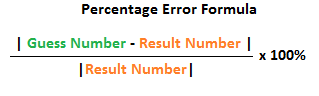# Why are basics important?

08/01/2019

A lot of my fellow data scientists ask me why they should know the statistics and basics behind their data science models. Frankly, one can survive in the data science world with knowing how to code some classification models. This example is a mistake I recently did which explains why understanding the basics is important.

Consider I have forecasted the temperature of some object to be 102 degrees centigrade. My friend actually checked the temperature and said that my prediction was 2 percent off. What is the actual temperature of the object?

Well, looking at the percentage of error, 2%, from 102 predicted value, I will calculate that the temperature is 100 degrees centigrade.Percentage error formula

But my friend actually was from a country where they use Fahrenheit instead of Centigrade, and when I said 102 degrees centigrade, he converted to 215.6 degrees fahrenheit.

So according to him, the error of 2% means 211.3 degrees fahrenheit ( which is 99.65 degrees centigrade)

So what is the actual temperature? 99.65 centigrade or 100 degrees centigrade?

There are four common levels of data measurement.
1. Nominal: Numbers representing nominal level data can be used only to classify or categorize.

2. Ordinal: In addition to the nominal level capabilities, ordinal-level measurement can be used to rank or order objects. The distances between consecutive numbers need not be the same.

3. Interval: Data in which the distances between consecutive numbers have meaning and the data are always numerical are called interval data. In addition, for interval data the zero point is a matter of convention or convenience and not a natural or fixed zero point.

4. Ratio: Ratio data have the same properties as interval data, but ratio data have an absolute zero, and the ratio of two numbers is meaningful.Each higher level of data not only be analyzed by any of the techniques used on lower levels of data but, in addition, can use other statistical techniques.

Therefore, ratio data can be analyzed by any statistical technique applicable to the other three levels of data plus some others.

Nominal data are the most limited data in terms of the types of statistical analysis that can be used with them.

On ordinal data, any analysis that can be done with nominal data can be used along some additional analysis.

With ratio data, ratio comparisons can be made, and any analysis that can be performed on nominal, ordinal, or interval data can be used.

Reference: Business Statistics for contemporary decision making - Ken Black

Temperature in centigrade scale is interval data as zero degrees centigrade is just a matter of convention. Error in percentage terms, like MAPE, are statistical methods which can be applied only on Ratio data, and not on interval data.

So 2% error in temperature is not the right way to calculate the error of my prediction.